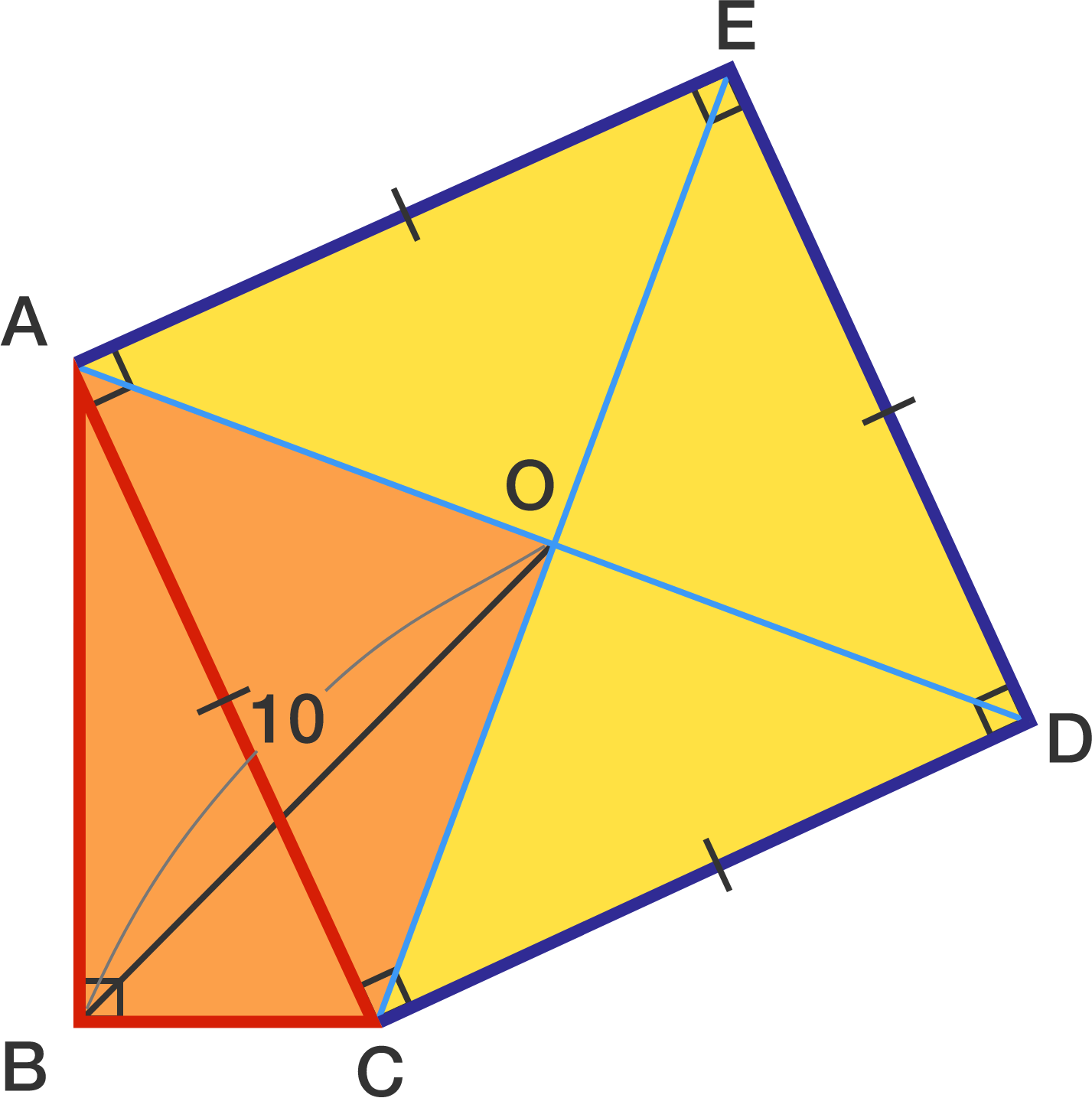# A geometry problem by Ajay Sambhriya

Geometry Level 3In triangle $ABC$, $\angle ABC = 90 ^ \circ$.
Square $ACDE$ with center $O$ is drawn externally on side $AC$ of the triangle.
If $OB = 10$, what is the area of quadrilateral $ABCO ?$

×